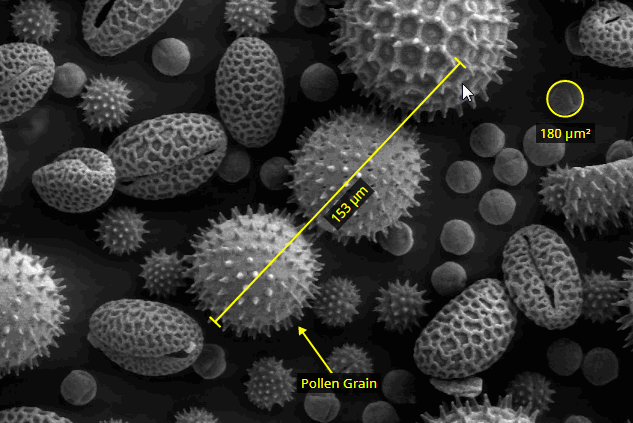/ Measure

# A React component for measuring & annotating images## react-measurements

A React component for measuring & annotating images.

## Usage

``````import React from "react";
import {
MeasurementLayer,
calculateDistance,
calculateArea
} from "react-measurements";

class App extends React.Component {
state = { measurements: [] };

render() {
return (
<div
style={{
position: "absolute",
width: "300px",
height: "300px",
backgroundColor: "#1a1a1a",
fontFamily: "sans-serif"
}}
>
<MeasurementLayer
measurements={this.state.measurements}
widthInPx={300}
heightInPx={300}
onChange={this.onChange}
measureLine={this.measureLine}
measureCircle={this.measureCircle}
/>
</div>
);
}

onChange = measurements => this.setState({ ...this.state, measurements });

measureLine = line => Math.round(calculateDistance(line, 100, 100)) + " mm";

measureCircle = circle =>
Math.round(calculateArea(circle, 100, 100)) + " mm²";
}
``````

## Scope

The component is currently read-only on mobile. A mouse is required to create and edit measurements.

## GitHub#### React.js Examples

A nice collection of often useful examples done in React.js.

Latest
Tag